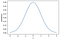# Statistical Distributions with Python Examples

A distribution provides a parameterised mathematical function that can be used to calculate the probability for any individual observation from the sample space.

The most common distributions are:

• Normal Distribution
• Student’s t-distribution
• Geometric distribution
• Bernoulli distribution
• Binomial distribution
• Poisson distribution
• Lognormal distribution

## Density Functions

Distribution are usually described in terms of their density functions:

• PDF (Probability Density Function) is used to calculate the likelihood of a given observation in a distribution and can be represented as follow.Gaussian PDF
• CDF (Cumulative Density Function) calculates the cumulative likelihood for the observation and all prior observations in the sample space. …

Data Analyst